# JonPan资料

### LeetCode-191 二进制位1的个数

LeetCode上第 191 号问题：编写一个函数，输入是一个无符号整数，返回其二进制表达式中数字位数为 ‘1’ 的个数。

``class Solution:     def hammingWeight(self, n):         res = 0         while n != 0:             res += 1             n &= (n - 1)         return res      def hammingWeight2(self, n):         res = 0         while n != 0:             res += (n & 1)             n = n >> 1         return res ``

### LeetCode-231 2的幂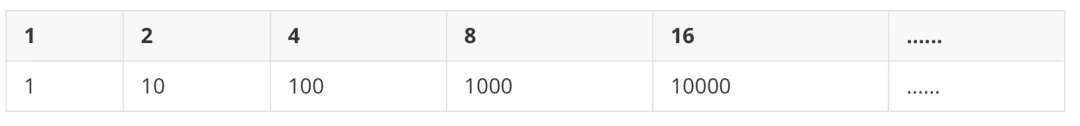``def isPowerOfTwo(n):         res = 0         while n != 0:             res += (n & 1)             n >>= 1         return res == 1 ``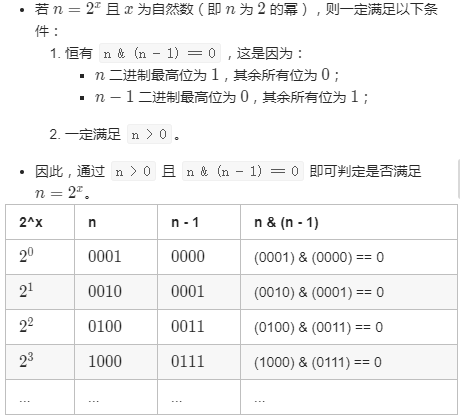``class Solution:     def isPowerOfTwo(self, n: int) -> bool:         return (n > 0) and ((n & (n - 1)) == 0) ``

### LeetCode-201. 闭区间范围内数字按位与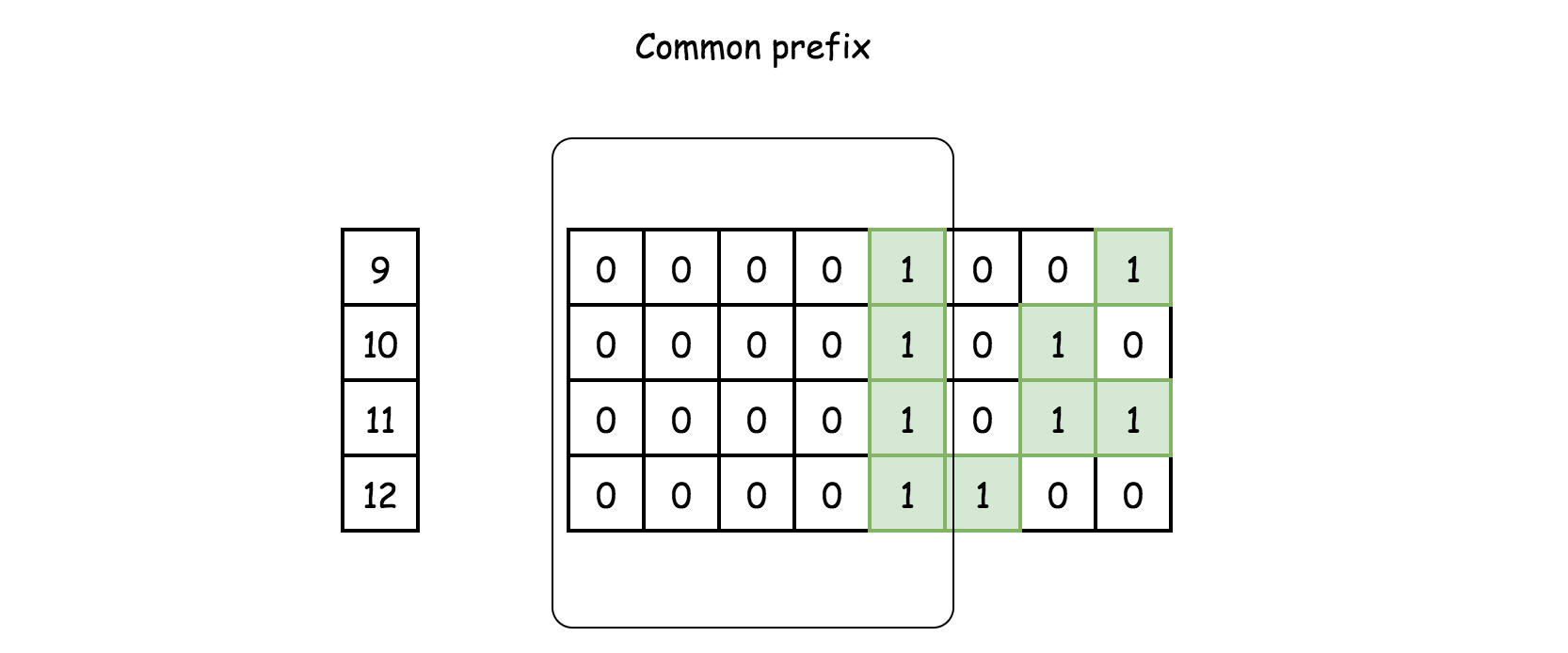1. 使用191的方法 `Brian Kernighan 算法` `n & (n-1) `
``class Solution:     def rangeBitwiseAnd(self, m: int, n: int) -> int:         while m < n:             # turn off rightmost 1-bit             n = n & (n - 1)         return m & n ``
1. 找m, n 的最高位1出现的位置 ， 如果不相等，则返回0，如果相等，则找公共前缀。B站视频-位运算练习【LeetCode】

### LeetCode-187.重复的DNA序列

``输入：s = "AAAAACCCCCAAAAACCCCCCAAAAAGGGTTT" 输出：["AAAAACCCCC", "CCCCCAAAAA"] ``
``# 普通解法 class Solution:     def findRepeatedDnaSequences(self, s: str) -> List[str]:         d = {}         for i in range(len(s) - 9):             k = s[i: i+10]             if k in d:                 d[k] = True             else:                 d[k] = False          return [*filter(lambda x: d[x], d)] ``

### LeetCode-36.只出现一次的数字

``输入: [2,2,1] 输出: 1 输入: [4,1,2,1,2] 输出: 4 ``

a b a⊕b
1 0 1
1 1 0
0 0 0
0 1 1
``class Solution:     def singleNumber(self, nums: List[int]) -> int:         # 非空数组暂时不用判断 		from functools import reduce     	return reduce(lambda a, b: a ^ b, nums) ``

### LeetCode-137.只出现一次的数字 II

``输入: [2,2,3,2] 输出: 3 输入: [0,1,0,1,0,1,99] 输出: 99 ``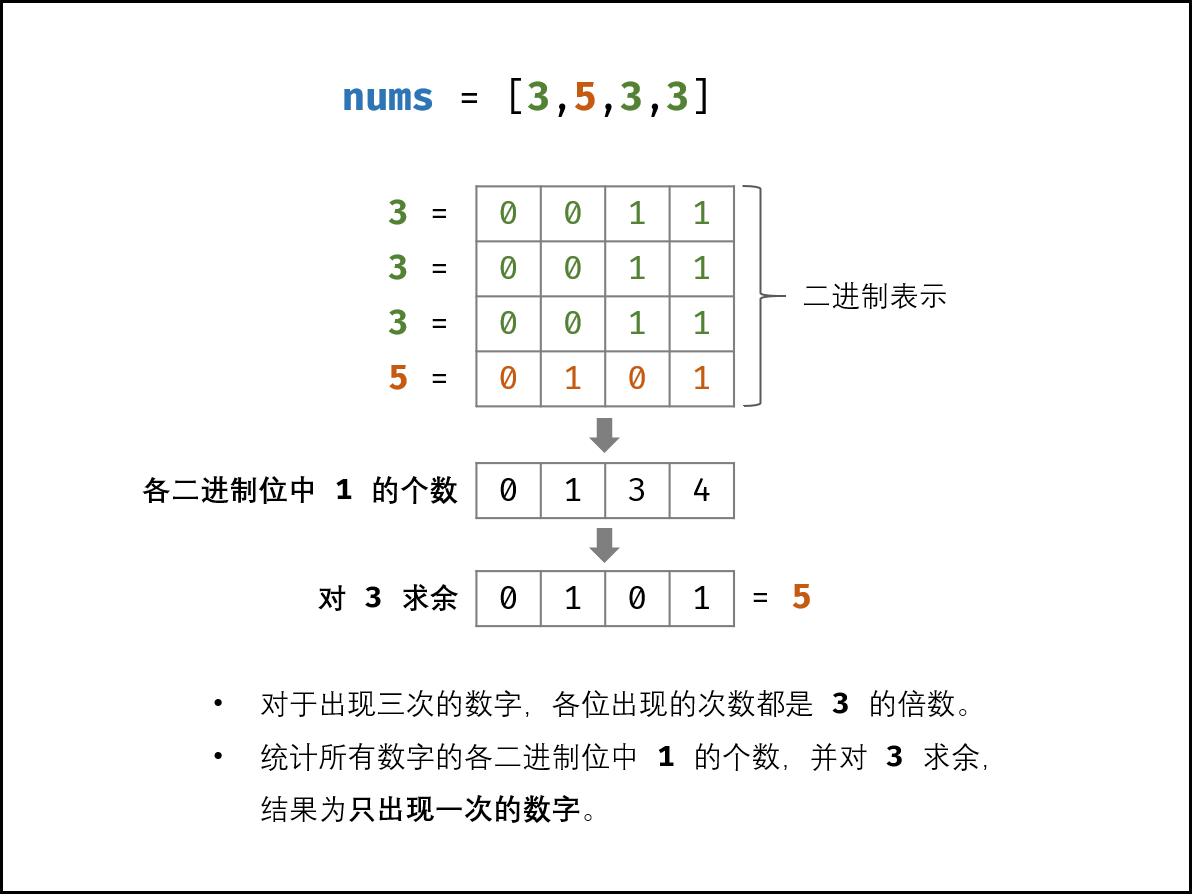3×(a+b+c)−(a+a+a+b+b+b+c)=2c 也可以应用在上一题

``## 普通解法 class Solution:     def singleNumber(self, nums):         return (3 * sum(set(nums)) - sum(nums)) // 2 ``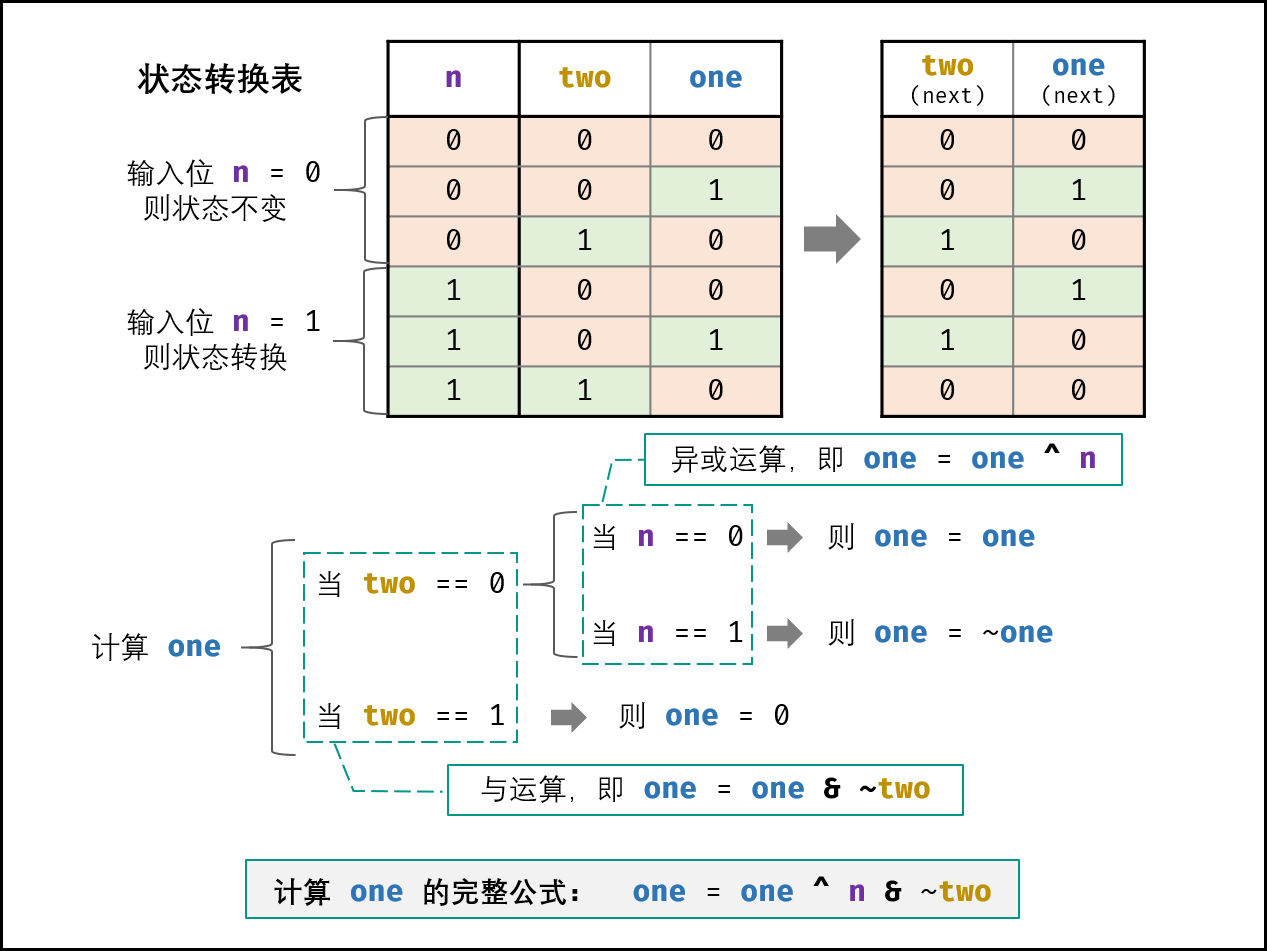1. 【自动机+位运算】最详细的推导过程

2. 图片来源：有限状态自动机 + 位运算，清晰图解-此题解清晰

``class Solution:     def singleNumber(self, nums: List[int]) -> int:         ones, twos = 0, 0         for num in nums:             ones = ones ^ num & ~twos             twos = twos ^ num & ~ones         return ones ``

### LeetCode-260. 只出现一次的数字 III

``输入: [1,2,1,3,2,5] 输出: [3,5] ``

1. 结果输出的顺序并不重要，对于上面的例子， `[5, 3]` 也是正确答案。
2. 你的算法应该具有线性时间复杂度。你能否仅使用常数空间复杂度来实现？

``class Solution:     def FindNumsAppearOnce(self, nums):         length = len(nums)         if length <= 0:             return nums         result = 0         # 先将所有数子异或得到一个值         for num in nums:             result ^= num         # 找到这个值最低位二进制位1的位置，根据这个位置来区分两个数组，分别异或求出只出现一次的数字         firstBitIndex = self.FindFirstBit(result)         n1, n2 = 0, 0         for num in nums:             if self.IsSameBit(num, firstBitIndex):                 n1 ^= num             else:                 n2 ^= num         return n1, n2      def FindFirstBit(self, num):         indexBit = 0         while num & 1 == 0:             indexBit += 1             num = num >> 1         return indexBit      def IsSameBit(self, num, indexBit):         num = num >> indexBit         return num & 1 ``
``# 解放2 class Solution2:     def FindNumsAppearOnce(self, nums):         length = len(nums)         if length <= 0:             return []          diff = 0         for i in nums:             diff ^= i              n1, n2 = 0, 0         minDiff = self.getMinDiff(diff)         for num in nums:             if minDiff & num == 0:                 n1 ^= num         n2 = diff ^ n1         return n1, n2      def getMinDiff(self, num):         # 保留一个低位是1的数字         # 取负号其实就是先取反，再加 1，需要 补码 的知识。最后再和原数相与就会保留最低位的 1。比如 1010，先取反是 0101，再加 1，就是 0110，再和 1010 相与，就是 0010 了         return num & (-num) ``

### 如何得到二进制位只有一个1的数，几种方法

diff &= -diff ; 这里 的做法。

diff = (diff & (diff – 1)) ^ diff; 这里 的做法

`n & (n - 1)` 的操作在 191 题 用过，它可以将最低位的 1 置为 0。比如 `1110`，先将最低位的 1 置为 0 就变成 `1100`，然后再和原数 `1110 `异或，就得到了` 0010`

diff = xor & ~(diff – 1) 这里 的做法

``mask=1 while((diff & mask)==0):     mask <<= 1 # mask 就是我们要构造的了 ``

### 参考资料

JonPan资料部分资料来自网络，侵权毕设源码联系删除

qklbishe.com区块链毕设代做网专注|以太坊fabric-计算机|java|毕业设计|代做平台 » JonPan资料